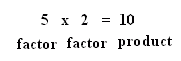Email us to get an instant 20% discount on highly effective K-12 Math & English kwizNET Programs!

#### Online Quiz (WorksheetABCD)

Questions Per Quiz = 2 4 6 8 10

### Grade 2 - Mathematics7.1 What is Multiplication?

 Explanation: Multiplication is repeated addition. Instead of adding many times, we just multiply the number by the number of times it appears.Here we can add 2 five times: 2 + 2 + 2 + 2 +2 = 10 You could also say 5 rows of two Or you can write that as a multiplication problemThe numbers that are multiplied 5 and 2 are called factors and 10 is called the product. Example: 3+3+3+3 This can be written as 3 x 4 In the above 3 and 4 are called factors and 12 is called the product. Answer : 12 Directions: Answer the following questions. Also write 20 multiplication facts of your own.
 Q 1: The product of 4 x 5 =202021 Q 2: What is the product of the multiplication 83 x 1 =83303880 Q 3: When two numbers are multiplied the answer is calledsumdifferenceproduct Q 4: The numbers that are being multiplied are calledsumfactorsproduct Q 5: What is the multiplication form for: 3+3+3+33 x 4 = 123 x 3 = 94 x 4 = 16 Q 6: What is the multiplication form for: 2 + 2 + 2 + 2 + 2 + 22 x 2 = 42 x 6 =122 x 5 = 10 Q 7: Multiplication is repeated addition where the addends are the same. Example 2+2+2+2+2=10 can also be written as 2x5=10truefalse Q 8: What are the factors of the multiplication 6 x 3 = 186 and 36 and 1818 and 3 Question 9: This question is available to subscribers only! Question 10: This question is available to subscribers only!